1 Introduction

In this vignette, we try to highlight further PopED features that may be useful. Only code related to
specific “new” features is described here in this vignette. These features (and more) are presented as r-scripts in the “examples” folder in the PopED installation directory located at:

system.file("examples", package="PopED")

You can view a list of the example files using the commands:

ex_dir <- system.file("examples", package="PopED")
list.files(ex_dir)

You can then open one of the examples (for example, ex.1.a.PK.1.comp.oral.md.intro.R) using the following code

file_name <- "ex.1.a.PK.1.comp.oral.md.intro.R"
ex_file <- system.file("examples",file_name,package="PopED")
file.copy(ex_file,tempdir(),overwrite = T)
file.edit(file.path(tempdir(),file_name))

The table below provides a check list of features for each of the available examples.

Features Ex1 Ex2 Ex3 Ex4 Ex5 Ex6 Ex7 Ex8 Ex9 Ex10 Ex11 Ex12 Ex13 Ex14 Ex15
Analytical model X X X X X X X - - - X X X X X
ODE model and doses X X - - - X - X X X - - - - -
Irregular dosing - - - - - - - - - - - - - - -
Full cov matrix W - - - - - - - - - - - - - - X
Inter-occ variability - - - - - - - - - - - - - X -
Discrete covariates - - - - - - X - - - X - - - -
Continuous covariates X X X X - X X X X X X X X X X
Multiple arms X - X X - - X X - - X X - X -
Multi response models - - X X - - - X - X - - - - -
Designs differ
across responses
- - - X - - - X - - - - - - -
Calculate precision
of derived parameters
- - - - - - - - - - - - - - -
Power calculation - - - - - - - - - - X - - - -
Include previous FIM - - - - - - - - - - X - - - -
Shrinkage/Bayesian FIM X X X X - - X - - X - - X - -
Discrete optimization X X X - - X - X - - - - - X -
Optimization
of more-group designs
(same response)
X - X X - - X X - - - - - X -
Different optimal
sampling times
between groups
- - - - - - - - - - - - - - -
Optimization with constraining
sampling times
X - X - - - - - - - - - - X -
Optimization of subjects
per group
- - - - - - - - - - - - - - -

2 Analytical solution PKPD model with 2 groups/arms; setup only

The full code for this example is available in ex.4.PKPD.1.comp.emax.R.

2.1 Define the PKPD model

Here we define a PKPD mode using analytical equations. The PK is a one compartment model with intravenus bolus administration and linear elimination. The PD is an ordinary Emax model driven by the PK concentrations. The expected output of each measurement (PK or PD) is given in the vector model_switch (see below for details).

library(PopED)
f_pkpdmodel <- function(model_switch,xt,parameters,poped.db){
with(as.list(parameters),{
y=xt
MS <- model_switch

# PK model
CONC = DOSE/V*exp(-CL/V*xt)

# PD model
EFF = E0 + CONC*EMAX/(EC50 + CONC)

y[MS==1] = CONC[MS==1]
y[MS==2] = EFF[MS==2]

return(list( y= y,poped.db=poped.db))
})
}

The error model also has to accommodate both response models.

## -- Residual Error function
## -- Proportional PK + additive PD
f_Err <- function(model_switch,xt,parameters,epsi,poped.db){
returnArgs <- do.call(poped.db$model$ff_pointer,list(model_switch,xt,parameters,poped.db))
y <- returnArgs[]
poped.db <- returnArgs[]

MS <- model_switch

prop.err <- y*(1+epsi[,1])

y[MS==1] = prop.err[MS==1]

return(list( y= y,poped.db =poped.db ))
}

Some of the parameters are assumed to have log-normal distributions in the population.

2.2 Define design and design space

We specify in the poped.db object the vector model_switch in order to assign the sampling times written in the vector xt to the PK (=1) or PD (=2) model.

poped.db <- create.poped.database(ff_fun=f_pkpdmodel,
fError_fun=f_Err,
fg_fun=f_etaToParam,
sigma=diag(c(0.15,0.015)),
bpop=c(CL=0.5,V=0.2,E0=1,EMAX=1,EC50=1),
d=c(CL=0.09,V=0.09,E0=0.04,EC50=0.09),
groupsize=20,
m=3,
xt=c( 0.33,0.66,0.9,5,0.1,1,2,5),
minxt=0,
maxxt=5,
bUseGrouped_xt=1,
model_switch=c(1,1,1,1,2,2,2,2),
a=list(c(DOSE=0),c(DOSE=1),c(DOSE=2)),
maxa=c(DOSE=10),
mina=c(DOSE=0))

The model predictions below show typical PK and PD profiles for three dose groups. The initial design, as shown in the poped.db object, consists of 3 arms with doses of 0, 1, and 2 mg; PK sampling times are 0.33, 0.66, 0.9, and 5 hours/days; PD sampling times are 0.1, 1, 2, and 5 hours/days. With model.names=c("PK","PD") one can name the outputs in the graph.

plot_model_prediction(poped.db,IPRED=TRUE,DV=TRUE,facet_scales="free",
separate.groups=TRUE,
model.names=c("PK","PD"))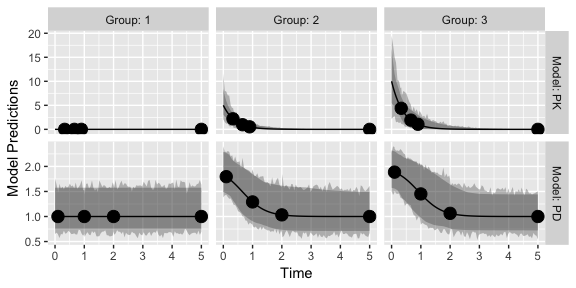3 ODE solution of PK model and multiple dose dosing scheme; design evaluation

The full code for this example is available in ex.9.PK.2.comp.oral.md.ode.compiled.R.

In this example, the deSolve library needs to be installed for computing solutions to a system of differential equations. For faster solutions one can use pre-compiled code using the Rcpp library.

library(Rcpp)
library(deSolve)

3.1 Define the system using ODEs

Here we define the two compartment model in R using deSolve notation

PK.2.comp.oral.ode <- function(Time, State, Pars){
with(as.list(c(State, Pars)), {
dA1 <- -KA*A1
dA2 <- KA*A1 + A3* Q/V2 -A2*(CL/V1+Q/V1)
dA3 <- A2* Q/V1-A3* Q/V2
return(list(c(dA1, dA2, dA3)))
})
}

Now we define the initial conditions of the ODE system A_ini with a named vector, in this case all compartments are initialized to zero c(A1=0,A2=0,A3=0). The dosing input is defined as a data.frame eventdat referring to the named compartment var = c("A1"), the specified dose_times and value=c(DOSE) dose amounts. Note that the covariates DOSE and the regimen TAU can differ by arm and be optimized (as shown in ex.1.a.PK.1.comp.oral.md.intro.R). For more information see the help pages for ?deSolve::ode and ?deSolve::events.

ff.PK.2.comp.oral.md.ode <- function(model_switch, xt, parameters, poped.db){
with(as.list(parameters),{
A_ini <- c(A1=0, A2=0, A3=0)
times_xt <- drop(xt)
dose_times = seq(from=0,to=max(times_xt),by=TAU)
eventdat <- data.frame(var = c("A1"),
time = dose_times,
value = c(DOSE), method = c("add"))
times <- sort(c(times_xt,dose_times))

# Here "PK.2.comp.oral.ode" is equivalent to the pre-compiled version "two_comp_oral_ode_Rcpp".
out <- ode(A_ini, times, PK.2.comp.oral.ode, parameters,
events = list(data = eventdat))#atol=1e-13,rtol=1e-13)
y = out[, "A2"]/(V1/Favail)
y=y[match(times_xt,out[,"time"])]
y=cbind(y)
return(list(y=y,poped.db=poped.db))
})
}

The parameter definition function should match the parameters used in the above two functions.

3.2 Define design and design space

We now create a PopED database. The ff_fun should now point to the function providing the solution of the ODE.

discrete_a <- cell(2,1)

poped.db <- create.poped.database(ff_fun="ff.PK.2.comp.oral.md.ode",
fg_fun="fg",
groupsize=20,
m=1,      #number of groups
bpop=c(CL=10,V1=100,KA=1,Q= 3.0, V2= 40.0, Favail=1),
d=c(CL=0.15^2,KA=0.25^2),
notfixed_bpop=c(1,1,1,1,1,0),
xt=c( 48,50,55,65,70,85,90,120),
minxt=0,
maxxt=144,
discrete_xt = list(0:144),
a=c(DOSE=100,TAU=24),
maxa=c(DOSE=1000,TAU=24),
mina=c(DOSE=0,TAU=8),
discrete_a = list(DOSE=seq(0,1000,by=100),TAU=8:24))

3.3 Plot intial design (just PRED)

We plot the initial design

plot_model_prediction(poped.db)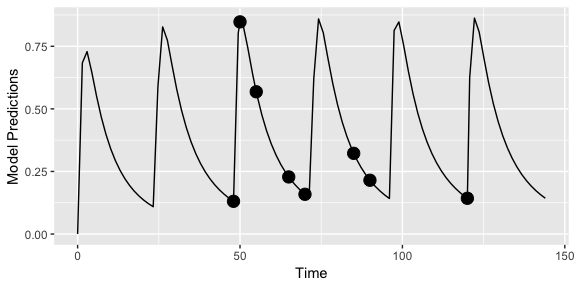3.4 Faster computations with Rcpp

We could also define the system using Rcpp, which will produce compiled code that should run faster (further examples in ex.2.c.warfarin.ODE.compiled.R). First we redefine the ODE system using Rcpp.

cppFunction('List two_comp_oral_ode_Rcpp(double Time, NumericVector A, NumericVector Pars) {
int n = A.size();
NumericVector dA(n);

double CL = Pars;
double V1 = Pars;
double KA = Pars;
double Q  = Pars;
double V2 = Pars;

dA = -KA*A;
dA = KA*A - (CL/V1)*A - Q/V1*A + Q/V2*A;
dA = Q/V1*A - Q/V2*A;
return List::create(dA);
}')

Next we solve this system of ODEs using deSolve.

Finally we create a poped database to use these functions by updating the previously created database.

poped.db.Rcpp <- create.poped.database(poped.db,
ff_fun="ff.PK.2.comp.oral.md.ode.Rcpp")

3.5 Design evaluation

We can compare the time for design evaluation with these two methods of describing the same model.

tic(); eval <- evaluate_design(poped.db); toc()
#> Elapsed time: 3.44 seconds.
tic(); eval <- evaluate_design(poped.db.Rcpp); toc()
#> Elapsed time: 1.232 seconds.

The difference is noticeable and gets larger for more complex and lengthy ODE models.

4 ODE solution for TMDD model with 2 outputs, arms with either single sc or iv dose, and different number of sampling times per arm

The full code for this example is available in ex.8.tmdd_qss_one_target_compiled.R.

Compile code using Rcpp for the TMDD model is as follows.

Define the parameters and the distributions of those parameters.

In the function that defines the dosing and derives the ODE solution, the discrete covariate SC_FLAG is used to give the dose either into A1 or A2, the sub-cutaneous or the IV compartment.

tmdd_qss_one_target_model_compiled <- function(model_switch,xt,parameters,poped.db){
with(as.list(parameters),{
y=xt

#The initialization vector for the compartment
A_ini <- c(A1=DOSE*SC_FLAG,
A2=DOSE*(1-SC_FLAG),
A3=0,
A4=R0)

#Set up time points for the ODE
times_xt <- drop(xt)
times <- sort(times_xt)
times <- c(0,times) ## add extra time for start of integration

# solve the ODE
out <- ode(A_ini, times, tmdd_qss_one_target_model_ode, parameters)#,atol=1e-13,rtol=1e-13)

# extract the time points of the observations
out = out[match(times_xt,out[,"time"]),]

# Match ODE output to measurements
RTOT = out[,"A4"]
CTOT = out[,"A2"]/V1
CFREE = 0.5*((CTOT-RTOT-KSSS)+sqrt((CTOT-RTOT-KSSS)^2+4*KSSS*CTOT))
COMPLEX=((RTOT*CFREE)/(KSSS+CFREE))
RFREE= RTOT-COMPLEX

y[model_switch==1]= RTOT[model_switch==1]
y[model_switch==2] =CFREE[model_switch==2]
#y[model_switch==3]=RFREE[model_switch==3]

return(list( y=y,poped.db=poped.db))
})
}

Finally we define the residual unexplained variability model.

4.1 Define the design

Two different sub-studies are defined, with different sampling times per arm - in terms of total number of samples and the actual times1. Due to this difference in numbers and the relatively complicated study design we define the sample times (xt) what each sample time will meansre (model_switch) and which samples should be taken at the same study time (G_xt) as matricies. Here three variables xt, model_switch, and G_xt are matrices with each row representing one arm, and the number of columns is the maximum number of samples (for all endpoints) in any of the arms (i.e., max(ni)). To be clear about which elements in the matriicies should be considered we specify the number of samples per arm by defining the vector ni in the create.poped.database() function.

xt <- zeros(6,30)
study_1_xt <- matrix(rep(c(0.0417,0.25,0.5,1,3,7,14,21,28,35,42,49,56),8),nrow=4,byrow=TRUE)
study_2_xt <- matrix(rep(c(0.0417,1,1,7,14,21,28,56,63,70,77,84,91,98,105),4),nrow=2,byrow=TRUE)
xt[1:4,1:26] <- study_1_xt
xt[5:6,] <- study_2_xt

model_switch <- zeros(6,30)
model_switch[1:4,1:13] <- 1
model_switch[1:4,14:26] <- 2
model_switch[5:6,1:15] <- 1
model_switch[5:6,16:30] <- 2

G_xt <- zeros(6,30)
study_1_G_xt <- matrix(rep(c(1:13),8),nrow=4,byrow=TRUE)
study_2_G_xt <- matrix(rep(c(14:28),4),nrow=2,byrow=TRUE)
G_xt[1:4,1:26] <- study_1_G_xt
G_xt[5:6,] <- study_2_G_xt

These can then be plugged into the normal poped.db setup.

poped.db.2 <- create.poped.database(ff_fun=tmdd_qss_one_target_model_compiled,
fError_fun=tmdd_qss_one_target_model_ruv,
fg_fun=sfg,
groupsize=rbind(6,6,6,6,100,100),
m=6,      #number of groups
sigma=c(0.04,0.0225),
bpop=c(CL=0.3,V1=3,Q=0.2,V2=3,FAVAIL=0.7,KA=0.5,VMAX=0,
KMSS=0,R0=0.1,KSSS=0.015,KDEG=10,KINT=0.05),
d=c(CL=0.09,V1=0.09,Q=0.04,V2=0.04,FAVAIL=0.04,
KA=0.16,VMAX=0,KMSS=0,R0=0.09,KSSS=0.09,KDEG=0.04,
KINT=0.04),
notfixed_bpop=c( 1,1,1,1,1,1,0,0,1,1,1,1),
notfixed_d=c( 1,1,1,1,1,1,0,0,1,1,1,1),
xt=xt,
model_switch=model_switch,
ni=rbind(26,26,26,26,30,30),
bUseGrouped_xt=1,
G_xt=G_xt,
a=list(c(DOSE=100, SC_FLAG=0),
c(DOSE=300, SC_FLAG=0),
c(DOSE=600, SC_FLAG=0),
c(DOSE=1000, SC_FLAG=1),
c(DOSE=600, SC_FLAG=0),
c(DOSE=1000, SC_FLAG=1)),
discrete_a = list(DOSE=seq(100,1000,by=100),
SC_FLAG=c(0,1)))

4.2 Plot model predictions

plot_model_prediction(poped.db.2,facet_scales="free")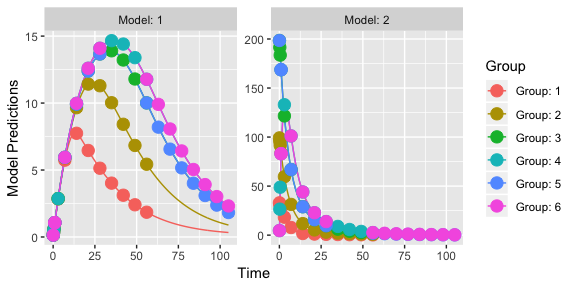4.3 Design evaluation

eval_2 <- evaluate_design(poped.db.2);
round(eval_2$rse) #> bpop bpop bpop bpop bpop bpop #> 2 2 2 3 3 5 #> bpop bpop bpop bpop D[1,1] D[2,2] #> 3 3 3 2 11 12 #> D[3,3] D[4,4] D[5,5] D[6,6] D[9,9] D[10,10] #> 22 20 24 19 12 13 #> D[11,11] D[12,12] SIGMA[1,1] SIGMA[2,2] #> 20 18 3 3 5 Model with continuous covariates The R code for this example is available in ex.12.covariate_distributions.R. Let’s assume that we have a model with a covariate included in the model description. Here we define a one-compartment PK model that uses allometric scaling with a weight effect on both clearance and volume of distribution. mod_1 <- function(model_switch,xt,parameters,poped.db){ with(as.list(parameters),{ y=xt CL=CL*(WT/70)^(WT_CL) V=V*(WT/70)^(WT_V) DOSE=1000*(WT/70) y = DOSE/V*exp(-CL/V*xt) return(list( y= y,poped.db=poped.db)) }) } par_1 <- function(x,a,bpop,b,bocc){ parameters=c( CL=bpop*exp(b), V=bpop*exp(b), WT_CL=bpop, WT_V=bpop, WT=a) return( parameters ) } Now we define a design. In this case one group of individuals, where we define the individuals’ typical weight as 70 kg (a=c(WT=70)). poped_db <- create.poped.database( ff_fun=mod_1, fg_fun=par_1, fError_fun=feps.add.prop, groupsize=50, m=1, sigma=c(0.015,0.0015), notfixed_sigma = c(1,0), bpop=c(CL=3.8,V=20,WT_CL=0.75,WT_V=1), d=c(CL=0.05,V=0.05), xt=c( 1,2,4,6,8,24), minxt=0, maxxt=24, bUseGrouped_xt=1, a=c(WT=70) ) We can create a plot of the model prediction for the typical individual plot_model_prediction(poped_db)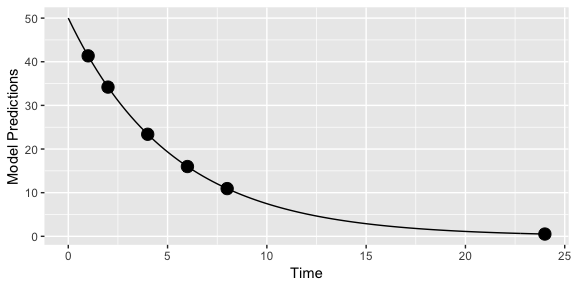And evaluate the initial design evaluate_design(poped_db) #> Problems inverting the matrix. Results could be misleading. #>$ofv
#>  -Inf
#>
#> $fim #> [,1] [,2] [,3] [,4] [,5] [,6] [,7] #> [1,] 65.8889583 -0.7145374 0 0 0.00000 0.00000 0.000 #> [2,] -0.7145374 2.2798156 0 0 0.00000 0.00000 0.000 #> [3,] 0.0000000 0.0000000 0 0 0.00000 0.00000 0.000 #> [4,] 0.0000000 0.0000000 0 0 0.00000 0.00000 0.000 #> [5,] 0.0000000 0.0000000 0 0 9052.31524 29.49016 1424.255 #> [6,] 0.0000000 0.0000000 0 0 29.49016 8316.09464 2483.900 #> [7,] 0.0000000 0.0000000 0 0 1424.25450 2483.90024 440009.144 #> #>$rse
#>    bpop    bpop    bpop    bpop     D[1,1]     D[2,2]
#>   3.247502   3.317107   0.000000   0.000000  21.026264  21.950179
#> SIGMA[1,1]
#>  10.061292

It is obvious that the covariate parameters can not be estimated according to this design calculation (RSE of bpop=0 and bpop=0). Why is that? Well, the calculation being done is assuming that every individual in the group has the same covariate (to speed up the calculation). This is clearly a poor prediction in this case!

5.1 Distribution of covariates

We can improve the computation by assuming a distribution of the covariate (WT) in the individuals in the study. We set groupsize=1, the number of groups to be 50 (m=50) and assume that WT is sampled from a normal distribution with mean=70 and sd=10 (a=as.list(rnorm(50, mean = 70, sd = 10)).

poped_db_2 <-
create.poped.database(
ff_fun=mod_1,
fg_fun=par_1,
groupsize=1,
m=50,
sigma=c(0.015,0.0015),
notfixed_sigma = c(1,0),
bpop=c(CL=3.8,V=20,WT_CL=0.75,WT_V=1),
d=c(CL=0.05,V=0.05),
xt=c(1,2,4,6,8,24),
minxt=0,
maxxt=24,
bUseGrouped_xt=1,
a=as.list(rnorm(50, mean = 70, sd = 10))
)
ev <- evaluate_design(poped_db_2)
round(ev$ofv,1) #>  43.1 round(ev$rse)
#>    bpop    bpop    bpop    bpop     D[1,1]     D[2,2]
#>          3          3         23         18         21         22
#> SIGMA[1,1]
#>         10

Here we see that, given this distribution of weights, the covariate effect parameters (bpop and bpop) would be well estimated.

However, we are only looking at one sample of 50 individuals. Maybe a better approach is to look at the distribution of RSEs over a number of experiments given the expected weight distribution.

nsim <- 30
rse_list <- c()
for(i in 1:nsim){
poped_db_tmp <-
create.poped.database(
ff_fun=mod_1,
fg_fun=par_1,
groupsize=1,
m=50,
sigma=c(0.015,0.0015),
notfixed_sigma = c(1,0),
bpop=c(CL=3.8,V=20,WT_CL=0.75,WT_V=1),
d=c(CL=0.05,V=0.05),
xt=c( 1,2,4,6,8,24),
minxt=0,
maxxt=24,
bUseGrouped_xt=1,
a=as.list(rnorm(50,mean = 70,sd=10)))
rse_tmp <- evaluate_design(poped_db_tmp)$rse rse_list <- rbind(rse_list,rse_tmp) } apply(rse_list,2,quantile) #> bpop bpop bpop bpop D[1,1] D[2,2] SIGMA[1,1] #> 0% 3.247565 3.317450 23.50614 18.02297 21.02295 21.94763 10.05802 #> 25% 3.249113 3.319010 28.22417 21.62949 21.02614 21.95268 10.06350 #> 50% 3.251032 3.320896 30.54414 23.41096 21.02677 21.95425 10.06516 #> 75% 3.273893 3.344324 33.55190 25.71118 21.02709 21.95566 10.06672 #> 100% 3.425038 3.498846 35.39505 27.12439 21.02999 21.96052 10.07182 Note, that the variance of the RSE of the covariate effect is in this case strongly correlated with the variance of the weight distribution (not shown). 6 Model with discrete covariates See ex.11.PK.prior.R. This has the covariate isPediatric to distinguish between adults and pediatrics. Alternatively, DOSE and TAU in the first example can be considered as discrete covariates but are not per se estimated but rather optimized. 7 Model with Inter-Occasion Variability The full code for this example is available in ex.14.PK.IOV.R. The IOV is introduced with bocc[x,y] in the parameter definition function as a matrix with the first argument x indicating the index for the IOV variances defined in docc below, and the second argument y denoting the occasion. This is used in the example to derive to different clearance values, i.e., CL_OCC_1 and CL_OCC_2 (think of some drug-drug interaction). sfg <- function(x,a,bpop,b,bocc){ parameters=c( CL_OCC_1=bpop*exp(b+bocc[1,1]), CL_OCC_2=bpop*exp(b+bocc[1,2]), V=bpop*exp(b), KA=bpop*exp(b), DOSE=a, TAU=a) return( parameters ) } These parameters can now be used in the model function to define the change in parameters between the occacions (here with the 7th dose in a one-compartment model with first order absorption). cppFunction( 'List one_comp_oral_ode(double Time, NumericVector A, NumericVector Pars) { int n = A.size(); NumericVector dA(n); double CL_OCC_1 = Pars; double CL_OCC_2 = Pars; double V = Pars; double KA = Pars; double TAU = Pars; double N,CL; N = floor(Time/TAU)+1; CL = CL_OCC_1; if(N>6) CL = CL_OCC_2; dA = -KA*A; dA = KA*A - (CL/V)*A; return List::create(dA); }' ) ff.ode.rcpp <- function(model_switch, xt, parameters, poped.db){ with(as.list(parameters),{ A_ini <- c(A1=0, A2=0) times_xt <- drop(xt) #xt[,,drop=T] dose_times = seq(from=0,to=max(times_xt),by=TAU) eventdat <- data.frame(var = c("A1"), time = dose_times, value = c(DOSE), method = c("add")) times <- sort(c(times_xt,dose_times)) out <- ode(A_ini, times, one_comp_oral_ode, c(CL_OCC_1,CL_OCC_2,V,KA,TAU), events = list(data = eventdat))#atol=1e-13,rtol=1e-13) y = out[, "A2"]/(V) y=y[match(times_xt,out[,"time"])] y=cbind(y) return(list(y=y,poped.db=poped.db)) }) } The within-subject variability variances (docc) are defined in the poped database as a 3-column matrix with one row per IOV-parameter, and the middle column giving the variance values. poped.db <- create.poped.database( ff_fun=ff.ode.rcpp, fError_fun=feps.add.prop, fg_fun=sfg, bpop=c(CL=3.75,V=72.8,KA=0.25), d=c(CL=0.25^2,V=0.09,KA=0.09), sigma=c(0.04,5e-6), notfixed_sigma=c(0,0), docc = matrix(c(0,0.09,0),nrow = 1), m=2, groupsize=20, xt=c( 1,2,8,240,245), minxt=c(0,0,0,240,240), maxxt=c(10,10,10,248,248), bUseGrouped_xt=1, a=list(c(DOSE=20,TAU=24),c(DOSE=40, TAU=24)), maxa=c(DOSE=200,TAU=24), mina=c(DOSE=0,TAU=24) ) 7.1 Create plot of the population predictions plot_model_prediction(poped.db, model_num_points = 300)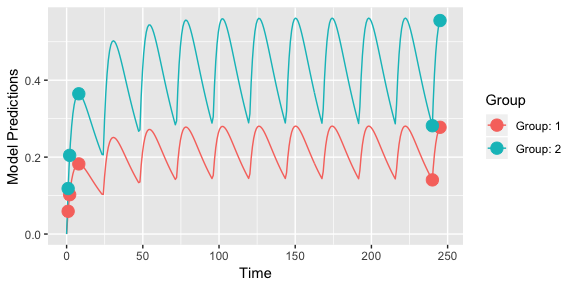7.2 visualize the IOV We can visualize the IOV by looking at an example individual. We see the PK profile changes at the 7th dose (red line) due to the change in clearance. library(ggplot2) set.seed(123) plot_model_prediction(poped.db, PRED=F,IPRED=F, separate.groups=T, model_num_points = 300, groupsize_sim = 1, IPRED.lines = T, alpha.IPRED.lines=0.6, sample.times = F ) + geom_vline(xintercept = 24*6,color="red")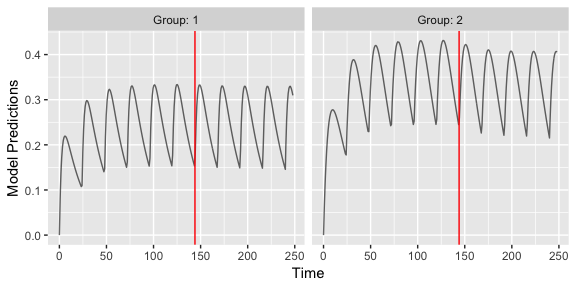7.3 Evaluate initial design ev <- evaluate_design(poped.db) round(ev$rse)
#>    bpop    bpop    bpop     D[1,1]     D[2,2]     D[3,3]
#>          6          9         11        106         43         63
#> D.occ[1,1]
#>         79

8 Full omega matrix

The full code for this example is available in ex.15.full.covariance.matrix.R.

The covd object is used for defining the covariances of the IIV variances (off-diagonal elements of the full variance-covariance matrix).

poped.db_with <-
create.poped.database(
ff_file="ff",
fg_file="sfg",
fError_file="feps",
bpop=c(CL=0.15, V=8, KA=1.0, Favail=1),
notfixed_bpop=c(1,1,1,0),
d=c(CL=0.07, V=0.02, KA=0.6),
covd = c(.03,.1,.09),
sigma=0.01,
groupsize=32,
xt=c( 0.5,1,2,6,24,36,72,120),
minxt=0,
maxxt=120,
a=70
)

What do the covariances mean?

(IIV <- poped.db_with$parameters$param.pt.val$d) #> [,1] [,2] [,3] #> [1,] 0.07 0.03 0.10 #> [2,] 0.03 0.02 0.09 #> [3,] 0.10 0.09 0.60 cov2cor(IIV) #> [,1] [,2] [,3] #> [1,] 1.0000000 0.8017837 0.4879500 #> [2,] 0.8017837 1.0000000 0.8215838 #> [3,] 0.4879500 0.8215838 1.0000000 They indicate a correlation of the inter-individual variabilities, here of ca. 0.8 between clearance and volume, as well as between volume and absorption rate. 8.1 Create plot of the model with variability library(ggplot2) p1 <- plot_model_prediction(poped.db,IPRED=TRUE)+ylim(0,12) p2 <- plot_model_prediction(poped.db_with,IPRED=TRUE) +ylim(0,12) gridExtra::grid.arrange(p1, p2, nrow = 1)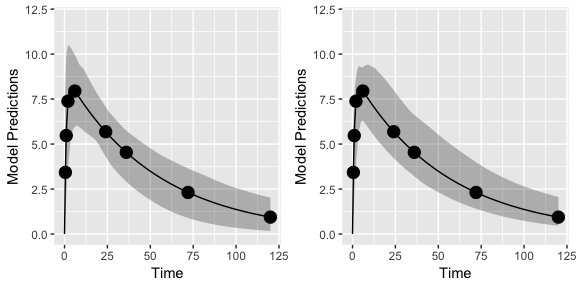8.2 Evaluate designs (with and without covariance matrix) ev1 <- evaluate_design(poped.db) ev2 <- evaluate_design(poped.db_with) round(ev1$ofv,1)
#>  52.4
round(ev1$rse,1) #> bpop bpop bpop D[1,1] D[2,2] D[3,3] #> 4.7 2.8 13.9 25.6 30.3 25.8 #> SIGMA[1,1] #> 11.2 round(ev2$ofv,1)
#>  85.9
round(ev2$rse,1) #> bpop bpop bpop D[1,1] D[2,2] D[3,3] #> 4.7 2.8 13.9 25.7 30.5 25.9 #> D_cov D_cov D_cov SIGMA[1,1] #> 30.8 41.5 30.7 11.2 Note, that the precision of all other parameters is barely affected by including the full covariance matrix. This is likely to be different in practice with much more ill-conditioned numerical problems. 8.3 Evaluate the same designs with full FIM (instead of reduced) ev1 <- evaluate_design(poped.db, fim.calc.type=0) ev2 <-evaluate_design(poped.db_with, fim.calc.type=0) round(ev1$ofv,1)
#>  55.5
round(ev1$rse,1) #> bpop bpop bpop D[1,1] D[2,2] D[3,3] #> 3.6 2.6 4.8 26.3 30.9 26.5 #> SIGMA[1,1] #> 12.4 round(ev2$ofv,1)
#>  88.2
round(ev2$rse,1) #> bpop bpop bpop D[1,1] D[2,2] D[3,3] #> 3.8 1.8 4.8 26.5 30.9 26.1 #> D_cov D_cov D_cov SIGMA[1,1] #> 31.0 41.7 31.1 12.3 9 Include a prior FIM, compute power to identify a parameter In this example you will learn how to incorporate prior knowledge to your current study. First the FIM obtained from an experiment in adulta is stored. Then this FIM is added to the current experiment in children. The full code for this example is available in ex.11.PK.prior.R. We define here the parameters for one compartment first order absorption model. Of note, isPediatric = x is the flag to switch between adults and pediatrics, and bpop=pedCL is the factor to multiply the adult clearance bpop to obtain the pediatric one. ## -- parameter definition function ## -- names match parameters in function ff ## -- note, covariate on clearance for pediatrics (using isPediatric x) sfg <- function(x,a,bpop,b,bocc){ parameters=c( V=bpop*exp(b), KA=bpop*exp(b), CL=bpop*exp(b)*bpop^x, # add covariate for pediatrics Favail=bpop, isPediatric = x, DOSE=a, TAU=a) return( parameters ) } Define design and design space for adults (Two arms, 5 time points, isPediatric = 0). As we want to pool the results, we also have to provide here the pedCL parameter. poped.db <- create.poped.database( ff_fun="ff", fg_fun="sfg", fError_fun="feps", bpop=c(V=72.8,KA=0.25,CL=3.75,Favail=0.9,pedCL=0.8), notfixed_bpop=c(1,1,1,0,1), d=c(V=0.09,KA=0.09,CL=0.25^2), sigma=c(0.04,5e-6), notfixed_sigma=c(0,0), m=2, groupsize=20, xt=c( 1,8,10,240,245), bUseGrouped_xt=1, a=list(c(DOSE=20,TAU=24),c(DOSE=40, TAU=24)), x=list(isPediatric = 0) ) Create plot of model without variability plot_model_prediction(poped.db, model_num_points = 300)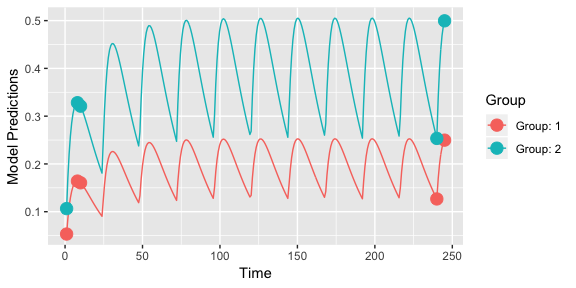To store the FIM from the adult design we evaluate the initial design (outAdult = evaluate_design(poped.db)) #> Problems inverting the matrix. Results could be misleading. #>$ofv
#>  -Inf
#>
#> $fim #> [,1] [,2] [,3] [,4] [,5] [,6] #> [1,] 0.05854391 -6.815269 -0.01531146 0 0.0000000 0.00000000 #> [2,] -6.81526942 2963.426688 -1.32113719 0 0.0000000 0.00000000 #> [3,] -0.01531146 -1.321137 37.50597895 0 0.0000000 0.00000000 #> [4,] 0.00000000 0.000000 0.00000000 0 0.0000000 0.00000000 #> [5,] 0.00000000 0.000000 0.00000000 0 1203.3695137 192.31775149 #> [6,] 0.00000000 0.000000 0.00000000 0 192.3177515 428.81459138 #> [7,] 0.00000000 0.000000 0.00000000 0 0.2184104 0.01919009 #> [,7] #> [1,] 0.000000e+00 #> [2,] 0.000000e+00 #> [3,] 0.000000e+00 #> [4,] 0.000000e+00 #> [5,] 2.184104e-01 #> [6,] 1.919009e-02 #> [7,] 3.477252e+03 #> #>$rse
#>   bpop   bpop   bpop   bpop    D[1,1]    D[2,2]    D[3,3]
#>  6.634931  8.587203  4.354792  0.000000 33.243601 55.689432 27.133255

It is obvious that we cannot estimate the pediatric covariate from adult data only; hence the warning message. You can also note the zeros in the 4th column and 4th row of the FIM indicating that pedCL cannot be estimated from the adult data.

We can evaluate the adult design without warning, by setting the pedCL parameter to be fixed (i.e., not estimated):

evaluate_design(create.poped.database(poped.db, notfixed_bpop=c(1,1,1,0,0)))
#> $ofv #>  29.70233 #> #>$fim
#>             [,1]        [,2]        [,3]         [,4]         [,5]
#> [1,]  0.05854391   -6.815269 -0.01531146    0.0000000   0.00000000
#> [2,] -6.81526942 2963.426688 -1.32113719    0.0000000   0.00000000
#> [3,] -0.01531146   -1.321137 37.50597895    0.0000000   0.00000000
#> [4,]  0.00000000    0.000000  0.00000000 1203.3695137 192.31775149
#> [5,]  0.00000000    0.000000  0.00000000  192.3177515 428.81459138
#> [6,]  0.00000000    0.000000  0.00000000    0.2184104   0.01919009
#>              [,6]
#> [1,] 0.000000e+00
#> [2,] 0.000000e+00
#> [3,] 0.000000e+00
#> [4,] 2.184104e-01
#> [5,] 1.919009e-02
#> [6,] 3.477252e+03
#>
#> $rse #> bpop bpop bpop D[1,1] D[2,2] D[3,3] #> 6.634931 8.587203 4.354792 33.243601 55.689432 27.133255 One obtains good estimates for all parameters for adults (<60% RSE for all). For pediatrics the covariate isPediatric = 1. We define one arm, 4 time points only poped.db.ped <- create.poped.database( ff_fun="ff", fg_fun="sfg", fError_fun="feps", bpop=c(V=72.8,KA=0.25,CL=3.75,Favail=0.9,pedCL=0.8), notfixed_bpop=c(1,1,1,0,1), d=c(V=0.09,KA=0.09,CL=0.25^2), sigma=c(0.04,5e-6), notfixed_sigma=c(0,0), m=1, groupsize=6, xt=c( 1,2,6,240), bUseGrouped_xt=1, a=list(c(DOSE=40,TAU=24)), x=list(isPediatric = 1) ) Create plot of the pediatric model without variability plot_model_prediction(poped.db.ped, model_num_points = 300)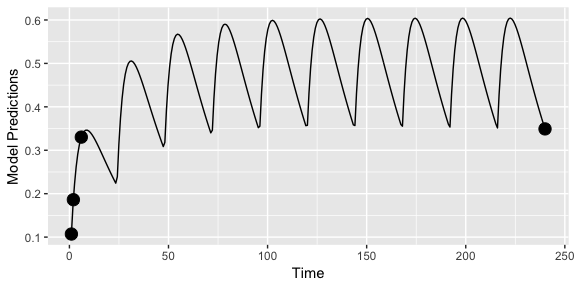Evaluate the design of the pediatrics study alone. evaluate_design(poped.db.ped) #> Problems inverting the matrix. Results could be misleading. #>$ofv
#>  -Inf
#>
#> $fim #> [,1] [,2] [,3] [,4] [,5] #> [1,] 0.007766643 -1.395981 -0.01126202 -0.05279073 0.0000000 #> [2,] -1.395980934 422.458209 -2.14666933 -10.06251250 0.0000000 #> [3,] -0.011262023 -2.146669 5.09936874 23.90329099 0.0000000 #> [4,] -0.052790734 -10.062512 23.90329099 112.04667652 0.0000000 #> [5,] 0.000000000 0.000000 0.00000000 0.00000000 141.1922923 #> [6,] 0.000000000 0.000000 0.00000000 0.00000000 53.7923483 #> [7,] 0.000000000 0.000000 0.00000000 0.00000000 0.7877291 #> [,6] [,7] #> [1,] 0.0000000 0.0000000 #> [2,] 0.0000000 0.0000000 #> [3,] 0.0000000 0.0000000 #> [4,] 0.0000000 0.0000000 #> [5,] 53.7923483 0.7877291 #> [6,] 58.0960085 0.3375139 #> [7,] 0.3375139 428.5254900 #> #>$rse
#>     bpop     bpop     bpop     bpop      D[1,1]      D[2,2]
#>  24.7208804  30.8495322   0.5200823  11.4275854 116.2309452 181.1977846
#>      D[3,3]
#>  77.2918849

Clearly the design has problems on its own.

We can add the prior information from the adult study and evaluate that design (i.e., pooling adult and pediatric data).

poped.db.all <- create.poped.database(
poped.db.ped,
prior_fim = outAdult$fim ) (out.all <- evaluate_design(poped.db.all)) #>$ofv
#>  34.96368
#>
#> $fim #> [,1] [,2] [,3] [,4] [,5] #> [1,] 0.007766643 -1.395981 -0.01126202 -0.05279073 0.0000000 #> [2,] -1.395980934 422.458209 -2.14666933 -10.06251250 0.0000000 #> [3,] -0.011262023 -2.146669 5.09936874 23.90329099 0.0000000 #> [4,] -0.052790734 -10.062512 23.90329099 112.04667652 0.0000000 #> [5,] 0.000000000 0.000000 0.00000000 0.00000000 141.1922923 #> [6,] 0.000000000 0.000000 0.00000000 0.00000000 53.7923483 #> [7,] 0.000000000 0.000000 0.00000000 0.00000000 0.7877291 #> [,6] [,7] #> [1,] 0.0000000 0.0000000 #> [2,] 0.0000000 0.0000000 #> [3,] 0.0000000 0.0000000 #> [4,] 0.0000000 0.0000000 #> [5,] 53.7923483 0.7877291 #> [6,] 58.0960085 0.3375139 #> [7,] 0.3375139 428.5254900 #> #>$rse
#>   bpop   bpop   bpop   bpop    D[1,1]    D[2,2]    D[3,3]
#>  6.381388  8.222819  4.354761 12.591940 31.808871 52.858399 25.601551

Clearly, the pooled data leads to much higher precision in parameter estimates compared to either study separately.

One can also obtain the power for estimating the pediatric difference in clearance (power in estimating bpop as different from 1).

evaluate_power(poped.db.all, bpop_idx=5, h0=1, out=out.all)
#> $ofv #>  34.96368 #> #>$fim
#>              [,1]       [,2]        [,3]         [,4]        [,5]
#> [1,]  0.007766643  -1.395981 -0.01126202  -0.05279073   0.0000000
#> [2,] -1.395980934 422.458209 -2.14666933 -10.06251250   0.0000000
#> [3,] -0.011262023  -2.146669  5.09936874  23.90329099   0.0000000
#> [4,] -0.052790734 -10.062512 23.90329099 112.04667652   0.0000000
#> [5,]  0.000000000   0.000000  0.00000000   0.00000000 141.1922923
#> [6,]  0.000000000   0.000000  0.00000000   0.00000000  53.7923483
#> [7,]  0.000000000   0.000000  0.00000000   0.00000000   0.7877291
#>            [,6]        [,7]
#> [1,]  0.0000000   0.0000000
#> [2,]  0.0000000   0.0000000
#> [3,]  0.0000000   0.0000000
#> [4,]  0.0000000   0.0000000
#> [5,] 53.7923483   0.7877291
#> [6,] 58.0960085   0.3375139
#> [7,]  0.3375139 428.5254900
#>
#> $rse #> bpop bpop bpop bpop D[1,1] D[2,2] D[3,3] #> 6.381388 8.222819 4.354761 12.591940 31.808871 52.858399 25.601551 #> #>$power
#>         Value      RSE power_pred power_want need_rse min_N
#> bpop   0.8 12.59194   51.01851         80 8.923519    14

We see that to clearly distinguish this parameter that one would need 14 children in the pediatric study (for 80% power at $$\alpha=0.05$$).

10 Design evaluation including uncertainty in the model parameters (robust design)

In this example the aim is to evaluate a design incorporating uncertainty around parameter values in the model. The full code for this example is available in ex.2.d.warfarin.ED.R. This illustration is one of the Warfarin examples from software comparison in: Nyberg et al.2.

10.1 Define the model parameters for use in poped.db

Adding 10% uncertainty to all fixed effects (not Favail)

bpop_vals <- c(CL=0.15, V=8, KA=1.0, Favail=1)
bpop_vals_ed <-
cbind(ones(length(bpop_vals),1)*4, # log-normal distribution
bpop_vals,
ones(length(bpop_vals),1)*(bpop_vals*0.1)^2) # 10% of bpop value
bpop_vals_ed["Favail",] <- c(0,1,0)
bpop_vals_ed
#>          bpop_vals
#> CL     4      0.15 0.000225
#> V      4      8.00 0.640000
#> KA     4      1.00 0.010000
#> Favail 0      1.00 0.000000

10.2 Define initial design and design space

Now bpop_vals_ed is a matrix and not only a vector and we can define the ED_samp_size=20 either here…

poped.db <-
create.poped.database(
ff_fun=ff,
fg_fun=sfg,
bpop=bpop_vals_ed,
notfixed_bpop=c(1,1,1,0),
d=c(CL=0.07, V=0.02, KA=0.6),
sigma=c(0.01,0.25),
groupsize=32,
xt=c( 0.5,1,2,6,24,36,72,120),
minxt=0,
maxxt=120,
a=70,
mina=0,
maxa=100,
ED_samp_size=20)

10.3 Design evaluation

Or provide the ED_samp_size as a parameter.

tic();evaluate_design(poped.db,d_switch=FALSE,ED_samp_size=20); toc()
#> $ofv #>  55.42646 #> #>$fim
#>             [,1]      [,2]      [,3]         [,4]        [,5]        [,6]
#> [1,] 17739.71944 21.032092 10.292809 0.000000e+00     0.00000  0.00000000
#> [2,]    21.03209 17.926273 -3.488356 0.000000e+00     0.00000  0.00000000
#> [3,]    10.29281 -3.488356 51.374424 0.000000e+00     0.00000  0.00000000
#> [4,]     0.00000  0.000000  0.000000 2.323781e+03    10.41360  0.03723339
#> [5,]     0.00000  0.000000  0.000000 1.041360e+01 19067.60658 11.75942235
#> [6,]     0.00000  0.000000  0.000000 3.723339e-02    11.75942 38.84190262
#> [7,]     0.00000  0.000000  0.000000 7.304572e+02  9671.38879 65.11480364
#> [8,]     0.00000  0.000000  0.000000 9.029499e+01   265.08323  2.93984016
#>             [,7]       [,8]
#> [1,]      0.0000    0.00000
#> [2,]      0.0000    0.00000
#> [3,]      0.0000    0.00000
#> [4,]    730.4572   90.29499
#> [5,]   9671.3888  265.08323
#> [6,]     65.1148    2.93984
#> [7,] 195403.8694 6605.44753
#> [8,]   6605.4475  476.08847
#>
#> $rse #> bpop bpop bpop D[1,1] D[2,2] D[3,3] #> 5.009423 2.974270 14.046395 29.761798 36.689605 26.751934 #> SIGMA[1,1] SIGMA[2,2] #> 31.352041 25.261525 #> Elapsed time: 0.127 seconds. We can see that the result, based on MC sampling, is somewhat variable with so few samples. tic();evaluate_design(poped.db,d_switch=FALSE,ED_samp_size=20); toc() #>$ofv
#>  55.4183
#>
#> $fim #> [,1] [,2] [,3] [,4] [,5] [,6] #> [1,] 17642.69415 20.920494 10.274939 0.000000e+00 0.00000 0.00000000 #> [2,] 20.92049 17.869670 -3.494226 0.000000e+00 0.00000 0.00000000 #> [3,] 10.27494 -3.494226 51.418805 0.000000e+00 0.00000 0.00000000 #> [4,] 0.00000 0.000000 0.000000 2.321305e+03 10.48148 0.03749921 #> [5,] 0.00000 0.000000 0.000000 1.048148e+01 19042.26959 11.78485456 #> [6,] 0.00000 0.000000 0.000000 3.749921e-02 11.78485 38.83521723 #> [7,] 0.00000 0.000000 0.000000 7.294105e+02 9672.53494 64.85942554 #> [8,] 0.00000 0.000000 0.000000 9.058182e+01 265.87291 2.95471273 #> [,7] [,8] #> [1,] 0.00000 0.000000 #> [2,] 0.00000 0.000000 #> [3,] 0.00000 0.000000 #> [4,] 729.41051 90.581818 #> [5,] 9672.53494 265.872915 #> [6,] 64.85943 2.954713 #> [7,] 194460.15973 6610.964912 #> [8,] 6610.96491 477.245341 #> #>$rse
#>    bpop    bpop    bpop     D[1,1]     D[2,2]     D[3,3]
#>   5.023172   2.979086  14.040869  29.778573  36.717642  26.754250
#> SIGMA[1,1] SIGMA[2,2]
#>  31.487815  25.277769
#> Elapsed time: 0.122 seconds.

11 Design evaluation for a subset of model parameters of interest (Ds optimality)

Ds-optimality is a criterion that can be used if one is interested in estimating a subset “s” of the model parameters as precisely as possible. The full code for this example is available in ex.2.e.warfarin.Ds.R.

11.1 Define initial design and design space

For Ds optimality we add the ds_index option to the create.poped.database function to indicate whether a paramter is interesting (=1) or not (=0). Moreover, we need to set the ofv_calc_type to 6 for computing the Ds optimality criterion, as it is set to 4 by default (for computing the log of the determiant of FIM). More details are available by running the command ?create.poped.database.

poped.db <-
create.poped.database(
ff_fun=ff,
fg_fun=sfg,
bpop=c(CL=0.15, V=8, KA=1.0, Favail=1),
notfixed_bpop=c(1,1,1,0),
d=c(CL=0.07, V=0.02, KA=0.6),
sigma=c(0.01,0.25),
groupsize=32,
xt=c( 0.5,1,2,6,24,36,72,120),
minxt=0,
maxxt=120,
a=70,
mina=0,
maxa=100,
ds_index=c(0,0,0,1,1,1,1,1), # size is number_of_non_fixed_parameters
ofv_calc_type=6) # Ds OFV calculation

11.2 Design evaluation

evaluate_design(poped.db)
#> $ofv #>  14534520 #> #>$fim
#>             [,1]      [,2]      [,3]         [,4]         [,5]        [,6]
#> [1,] 17141.83891 20.838375 10.011000 0.000000e+00     0.000000  0.00000000
#> [2,]    20.83837 17.268051 -3.423641 0.000000e+00     0.000000  0.00000000
#> [3,]    10.01100 -3.423641 49.864697 0.000000e+00     0.000000  0.00000000
#> [4,]     0.00000  0.000000  0.000000 2.324341e+03     9.770352  0.03523364
#> [5,]     0.00000  0.000000  0.000000 9.770352e+00 19083.877564 11.72131703
#> [6,]     0.00000  0.000000  0.000000 3.523364e-02    11.721317 38.85137516
#> [7,]     0.00000  0.000000  0.000000 7.268410e+02  9656.158553 64.78095548
#> [8,]     0.00000  0.000000  0.000000 9.062739e+01   266.487127  2.94728469
#>              [,7]        [,8]
#> [1,]      0.00000    0.000000
#> [2,]      0.00000    0.000000
#> [3,]      0.00000    0.000000
#> [4,]    726.84097   90.627386
#> [5,]   9656.15855  266.487127
#> [6,]     64.78096    2.947285
#> [7,] 192840.20092 6659.569867
#> [8,]   6659.56987  475.500111
#>
#> \$rse
#>    bpop    bpop    bpop     D[1,1]     D[2,2]     D[3,3]
#>   5.096246   3.031164  14.260384  29.761226  36.681388  26.748640
#> SIGMA[1,1] SIGMA[2,2]
#>  32.011719  25.637971

12 Shrinkage

The full code for this example is available in “ex.13.shrinkage.R”.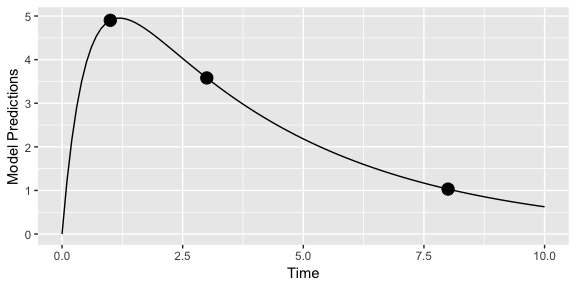To evaluate the estimation quality of the individual random effects in the model (the b’s) we use the function shrinkage().

shrinkage(poped.db)
#> # A tibble: 3 x 5
#>   d d d type       group
#>    <dbl>  <dbl>  <dbl> <chr>      <chr>
#> 1  0.504  0.367  0.424 shrink_var grp_1
#> 2  0.295  0.205  0.241 shrink_sd  grp_1
#> 3  0.710  0.303  0.206 se         grp_1

The output shows us the expected shrinkage on the variance scale ($$shrink_{var}=1-var(b_j)/D(j,j)$$) and on the standard deviation scale ($$shrink_{sd}=1-sd(b_j)/sqrt(D(j,j))$$), as well as the standard errors of the $$b_j$$ estimates.

13 Further examples (to be implemented)

• Espresso design
• Handling BLQ data
• Irregular dosing more complex: e.g. switching between s.c. and i.v. within one arm.
• Constraining the optimization to different allowed sampling times for each group
• Constraining the optimization to different allowed sampling times for each response
• Keep some sampling time fixed (they will be automatically part of the optimal design protocol)
• Handling derived outputs
• Symbolic differentiation

1. Study 1 and 2 from table 2 in: Gibiansky, L., Gibiansky, E., & Bauer, R. (2012). Comparison of Nonmem 7.2 estimation methods and parallel processing efficiency on a target-mediated drug disposition model. Journal of Pharmacokinetics and Pharmacodynamics, 39(1), 17–35. https://doi.org/10.1007/s10928-011-9228-y

2. Nyberg, J., Bazzoli, C., Ogungbenro, K., Aliev, A., Leonov, S., Duffull, S., Hooker, A.C. and Mentré, F. (2014). Methods and software tools for design evaluation for population pharmacokinetics-pharmacodynamics studies. British Journal of Clinical Pharmacology, 79(1), 1–32. https://doi.org/10.1111/bcp.12352